International
Tables for
Crystallography
Volume C
Mathematical, physical and chemical tables
Edited by E. Prince

International Tables for Crystallography (2006). Vol. C, ch. 8.2, pp. 689-691

## Section 8.2.2. Robust/resistant methods

E. Princea and D. M. Collinsb

aNIST Center for Neutron Research, National Institute of Standards and Technology, Gaithersburg, MD 20899, USA, and bLaboratory for the Structure of Matter, Code 6030, Naval Research Laboratory, Washington, DC 20375-5341, USA

### 8.2.2. Robust/resistant methods

| top | pdf |

Properly weighted least squares gives the best linear estimate for a very broad range of distributions of random errors in the data and the maximum-likelihood estimate if that error distribution is normal or Gaussian. But the best linear estimator may nevertheless not be a very good one, and the error distribution may not be well known. It is therefore important to address the question of how good an estimation procedure may be when the conditions for which it is designed may not be satisfied. Refinement procedures may be classified according to the extent that they possess two properties known as robustness and resistance. A procedure is said to be robust if it works well for a broad range of error distributions and resistant if its results are not strongly affected by fluctuations in any small subset of the data. Because least squares is a linear estimator, the influence of any single data point on the parameter estimates increases without limit as the difference between the observation and the model increases. It therefore works poorly if the actual error distribution contains large deviations with a frequency that substantially exceeds that expected from a normal distribution. Further, it has the undesirable property that it will make the fit of a few wildly discrepant data points better by making the fit of many points a little worse. Least squares is therefore neither robust nor resistant.

Tukey (1974) has listed a number of properties a procedure should have in order to be robust and resistant. Because least squares works well when the error distribution is normal, the procedure should behave like least squares for small deviations whose distribution is similar to the normal distribution. It should de-emphasize large differences between the model and the data, and it should connect these extremes smoothly. A procedure suggested by Tukey was applied to crystal structure refinement by Nicholson, Prince, Buchanan & Tucker (1982). It corresponds to a fitting function ρ(Δ) [equation (8.2.1.5)] of the form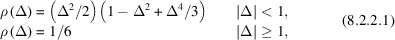where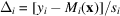, and s is a resistant measure of scale.

In order to see what is meant by a resistant measure, consider a large sample of observations,, with a normal distribution. The sample mean,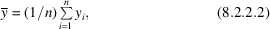is an unbiased estimate of the population mean. Contamination of the sample by a small number of observations containing large, systematic errors, however, would have a large effect on the estimate. The median value ofis also an unbiased estimate of the population mean, but it is virtually unaffected by a few contaminating points. Similarly, the sample variance,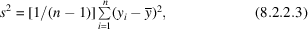is an unbiased estimate of the population variance, but, again, it is strongly affected by a few discrepant points, whereas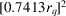, where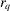is the interquartile range, the difference between the first and third quartile observations, is an estimate of the population variance that is almost unaffected by a small number of discrepant points. The median and the interquartile range are thus resistant quantities that can be used to estimate the mean and variance of a population distribution when the sample may contain points that do not belong to the population. A value of the scale parameter,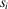, for use in evaluating the quantities in (8.2.2.1), that has proved to be useful is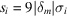, where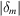represents the median value of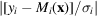, the median absolute deviation, or MAD.

Implementation of a procedure based on the function given in (8.2.2.1)involves modification of the weights used in each cycle by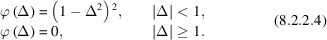Because of this weight modification, the procedure is sometimes referred to as iteratively reweighted least squares'. It should be recognized, however, that the function that is minimized is more complex than a sum of squares. In a strict application of the Gauss–Newton algorithm (see Section 8.1.3) to the minimization of this function, each term in the summations to form the normal-equations matrix contains a factor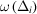, where ω(Δ) = d2ρ/dΔ2 = 1 − 6Δ2 + 5Δ4. This factor actually gives some data points a negative effective weight', because the sum is actually reduced by making the fit worse. The inverse of this normal-equations matrix is not an estimate of the variance–covariance matrix; for that the unmodified weights, equal to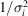, must be used, but, because more discrepant points have been deliberately down weighted relative to the ideal weights, the variances are, in general, underestimated. A recommended procedure (Huber, 1973; Nicholson et al., 1982) is to calculate the normal-equations matrix using the unmodified weights, invert that matrix, and premultiply by an estimate of the variance of the residuals (Section 8.4.1) using modified weights and (np) degrees of freedom. Huber showed that this estimate is biased low, and suggests multiplication by a number,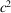, greater than one, and given by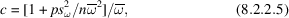where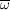is the mean value, and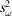is the variance ofover the entire data set. The conditions under which this expression is derived are not well satisfied in the crystallographic problem, but, if n/p is large andis not too much less than one, the value of c will be close to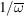.plays the role of a `variance efficiency factor'. That is, the variances are approximately those that would be achieved with a least-squares fit to a data set with normally distributed errors that contained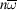data points.

Robust/resistant methods have been discussed in detail by Huber (1981), Belsley, Kuh & Welsch (1980), and Hoaglin, Mosteller & Tukey (1983). An analysis by Wilson (1976) shows that a fitting procedure gives unbiased estimates if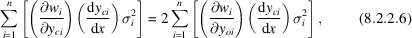where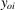and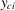are the observed and calculated values of, respectively. Least squares is the case where all terms on both sides of the equation are equal to zero; the weights are fixed. In maximum-likelihood estimation or robust/resistant estimation, the effective weights are functions of the deviation, causing possible introduction of bias. Equation (8.2.2.6), however, suggests that the estimates will still be unbiased if the sums on both sides are zero, which will be the case if the error distribution and the weight modification function are both symmetric about Δ = 0.

Note that the fact that two different weighting schemes applied to the same data lead to different values for the estimate does not necessarily imply that either value is biased. As long as the observations represent unbiased estimates of the values of the model functions, any weighting scheme gives unbiased estimates of the model parameters, although some weighting schemes will cause those estimates to be more precise than others will. Bias can be introduced if a procedure systematically causes fluctuations in one direction to be weighted more heavily than fluctuations in the other. For example, in the Rietveld method (Chapter 8.6), the observations are counts of quanta, which are subject to fluctuation according to the Poisson distribution, where the probability of observing k counts per unit time is given by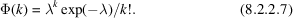The mean and the variance of this p.d.f. are both equal to λ, so that the ideal weighting should have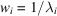. However, λi is not known a priori, and must be estimated. The usual procedure is to takeas an estimate of λi, but this is an unbiased estimate only asymptotically for large k (Box & Tiao, 1973), and, furthermore, causes observations that have negative, random errors to be weighted more heavily than observations that have positive ones. This correlation can be removed by using, after a preliminary cycle of refinement,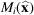as an estimate of. This might seem to have the effect of making the weights dependent on the calculated values, so that the right-hand side of (8.2.2.6)is no longer zero, but this applies only if the weights are changed during the refinement. There is thus no conflict with the result in (equation 8.1.2.9). In practice, in any case, many other sources of uncertainty are much more important than any possible bias that could be introduced by this effect.

### References

Belsley, D. A., Kuh, E. & Welsch, R. E. (1980). Regression diagnostics. New York: John Wiley.
Box, G. E. P. & Tiao, G. C. (1973). Bayesian inference in statistical analysis. Reading, MA: Addison-Wesley.
Hoaglin, D. C., Mosteller, M. & Tukey, J. W. (1983). Understanding robust and exploratory data analysis. New York: John Wiley.
Huber, P. J. (1973). Robust regression: asymptotics, conjectures and Monte Carlo. Ann. Stat. 1, 799–821.
Huber, P. J. (1981). Robust statistics. New York: John Wiley.
Nicholson, W. L., Prince, E., Buchanan, J. & Tucker, P. (1982). A robust/resistant technique for crystal structure refinement. Crystallographic statistics: progress and problems, edited by S. Rameseshan, M. F. Richardson & A. J. C. Wilson, pp. 220–263. Bangalore: Indian Academy of Sciences.
Tukey, J. W. (1974). Introduction to today's data analysis. Critical evaluation of chemical and physical structural information, edited by D. R. Lide & M. A. Paul, pp. 3–14. Washington: National Academy of Sciences.
Wilson, A. J. C. (1976). Statistical bias in least-squares refinement. Acta Cryst. A32, 994–996.